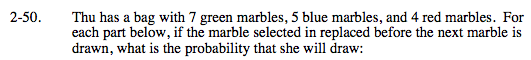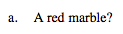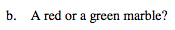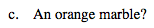### Home > MC2 > Chapter 2 > Lesson 2.2.1 > Problem2-50

2-50.Remember that probability can be written as a fraction:

$\frac{\text{\# of marbles we're looking for}}{\text{Total \# of marbles}}$

How many red marbles are there?
How many total marbles?See part (a).

$\frac{11}{16}$See part (a).

Explore the problem with the eTool below.
Click the link at right for the full version of the eTool: MC2 2-50 HW eTool